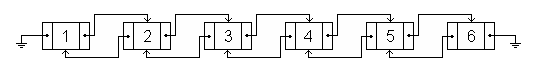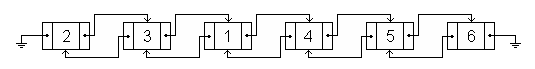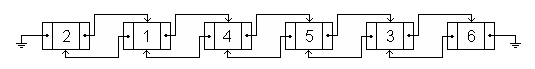### COCI 2006/2007, Contest #3

Mirko received a birthday present from his aunt in the US – a brand-new doubly-linked list (an example of which is shown in the figure below). The list contains N nodes numbered 1 through N. Two types of moves can be done on the list:

• A) Move node X in front of node Y.
• B) Move node X after node Y.An example of a list with 6 nodes.The list after the move "A 1 4".The list after another move, "B 3 5".

Mirko played with his new toy for hours, writing down each move on a piece of paper so that he can reconstruct the list's initial state (nodes 1 through N in order from left to right).

When he decided to reconstruct the list, Mirko was astonished to find that there is no easy way to invert the moves and restore the list's initial state. Mirko cannot know where node X was prior to each move, only where it ended up.

Seeing how Mirko is still recovering from the shock, write a program that finds a minimal sequence of moves that restored the list's initial state from Mirko's logs.

### Input

The first line of input contains two integers N and K (2 ≤ N ≤ 500 000, 0 ≤ M ≤ 100 000), the number of nodes and the number of moves made by Mirko.

Each of the next M rows contains a description of a single move made by Mirko – the type of move ('A' or 'B') and two integers X and Y.

### Output

Output the minimum number of moves (call this number K) on the first line.

Each of the next K lines should contain a description of a single move in the same format as in the input.

Note: The sequence need not be unique.

### Scoring

If both the number K and the sequence of moves are correct, your program will score full points on the test case.

If your program outputs the correct number K and does not output the sequence of moves, or the sequence of moves is incorrect, you will get 60% of the points for that test case.

```2 1
A 2 1
```

```1
A 1 2
```

```4 3
B 1 2
A 4 3
B 1 4
```

```2
A 1 2
B 4 3
```

```6 5
A 1 4
B 2 5
B 4 2
B 6 3
A 3 5
```

### Output

```3
A 4 5
B 6 5
A 2 3
```

Point Value: 20 (partial)
Time Limit: 1.00s
Memory Limit: 32M